# Bar Bending Schedule for RCC Box Culvert | What is Box Culvert | Advantages of RCC Box Culvert | Box Culvert Quantity Calculation:

In this Article today we will talk about the Bar Bending Schedule for RCC Box Culvert | Box Culvert Quantity Calculation | Advantages of RCC Box Culvert | Box Culvert Estimate | Reinforcement Details of RCC Box Culvert | BBS of Box Culvert

Box culverts are made up of concrete and especially, RCC (Reinforced Concrete). The most challenging part in constructing a box culvert is that dry surface is needed for installing it. However, due to the strength of the concrete floor, water direction can be changed when a large amount of water is expected. This feature makes box culverts, one of the most commonly found types of the culvert. Bar Bending Schedule for RCC Box Culvert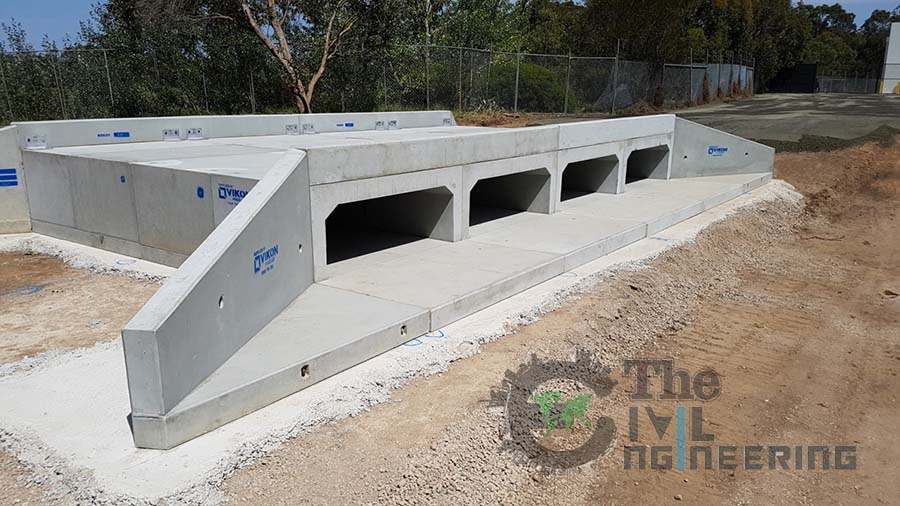Box Culverts are economical for the reasons mentioned below:

• The box culvert is a rigid frame structure and very simple in construction
• It is Suitable for non-perennial streams where scrub depth is not significant but the soil is weak. Box Culvert Quantity Calculation
• The bottom slab of the box culvert reduces pressure on the soil.
• Box culverts are economical due to their rigidity and monolithic action and separate foundations are not required. Box Culvert Quantity Calculation
• It is used in special cases, weak foundation. Box Culvert Quantity Calculation

### Bar Bending Schedule of RCC Box Culvert:

In the reinforcement, there are top slab, bottom slab and outside walls. Box culvert typical section (6×7), where 6 are inside length and 7 is inside height. Top slab has top main bar and bottom main bar. Dots signify distribution bars. Bottom slab has top main bar and bottom main bar. Dots signify distribution bars. There’re outside walls with vertical main bars. Dots indicate distribution bars. Advantages of RCC Box Culvert

### GIVEN DATA: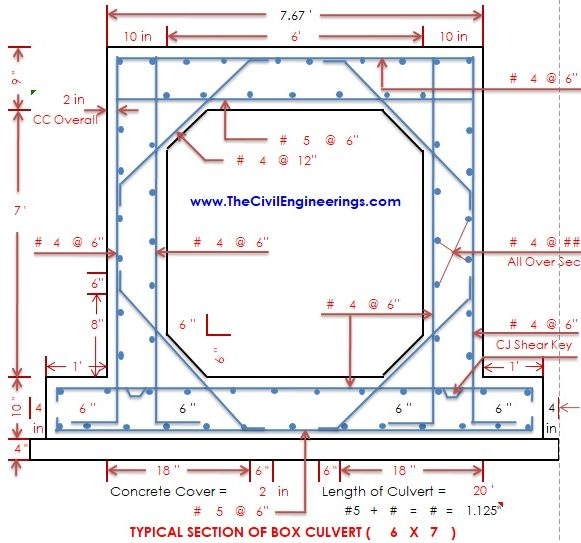#### RCC Box Culvert:

Typical Section of Box Culvert = 4’ x 7’

Length of RCC Box Culvert = 20’

Length of Top Slab = 7.67’

Length of Bottom Slab = 7.67 + 1 + 1 = 9.67’

Overall Concrete Cover: 2” = 0.17’

Bend: 3” = 0.25’

#5 = 0.625” Advantages of RCC Box Culvert

#4 = 0.5”

#9 = 1.125” = 0.09375’

#### Top RCC Slab of Culvert:

Top Main Bars: #4 @ 6” C/C

Bottom Main Bars: #5 @ 6” C/C

Distribution Bars: #4 @ 12” C/C

#### Bottom RCC Slab of Culvert:

Top Main Bars: #4 @ 6” C/C

Bottom Main Bars: #5 @ 6” C/C

Distribution Bars: #4 @ 12” C/C

#### Outside RCC Walls of Culvert:

Vertical Bars: #4 @ 6” C/C

Distribution Bars: #4 @ 12” C/C

#### Concrete Fillet of Culvert:

Haunch Bars: #4 @ 12” C/C Advantages of RCC Box Culvert

### SOLUTION:

#### 1. Top Slab Top Main Bars of RCC Box Culvert: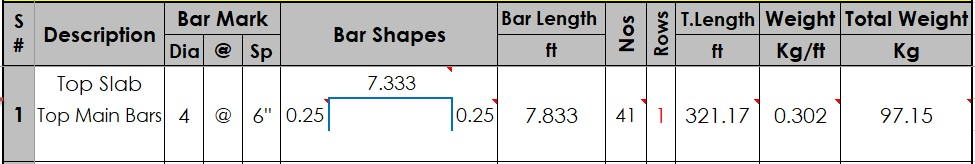Cut Length of Top Main Bars = Length of Top Slab – (2 x CC) + (2 x Bend Length)

Cut Length of Top Main Bars = 7.67’ – (2 x 0.17’) + (2 x 0.25’)

Cut Length of Top Main Bars = 7.67’- 0.34 + 0.50

Cut Length of Top Main Bars = 7.833’

Box Culvert Estimate

No’s of Top Main Bars = (Length of Culvert – 2xCC) / Spacing +1

No’s of Top Main Bars = (20’ – 2×0.17) / 0.5 +1

No’s of Top Main Bars = 19.66 / 0.5 + 1 = 39.32 + 1

No’s of Top Main Bars = 40.32 = Say 41 No’s

Box Culvert Estimate

Total Length of Top Main Bars = Cut Length x No’s of Bars

Total Length of Top Main Bars = 7.833’ x 41

Total Length of Top Main Bars = 321.153

Box Culvert Estimate

Weight of Top Main Bars = d2/52.896 x Total Length (where d is in # Nos)

Weight of Top Main Bars = 42/52.896 x 321.153

Weight of Top Main Bars = 97.15 Kg

Box Culvert Estimate

#### 2. Top Slab Bottom Main Bars of RCC Box Culvert: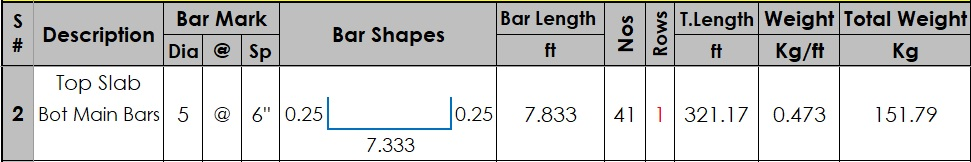Cut Length of Bottom Mai Bars = Length of Top Slab – (2 x CC) + (2 x Bend Length)

Cut Length of Bottom Top Main Bars = 7.67’ – (2 x 0.17’) + (2 x 0.25’)

Cut Length of Bottom Main Bars = 7.67’- 0.34 + 0.50

Cut Length of Bottom Main Bars = 7.833’

Box Culvert Estimate

No’s of Bottom Main Bars = (Length of Culvert – 2xCC) / Spacing +1

No’s of Bottom Main Bars = (20’ – 2×0.17) / 0.5 +1

No’s of Bottom Main Bars = 19.66 / 0.5 + 1 = 39.32 + 1

No’s of Bottom Main Bars = 40.32 = Say 41 No’s

Reinforcement Details of RCC Box Culvert

Total Length of Bottom Main Bars = Cut Length x No’s of Bars

Total Length of Bottom Main Bars = 7.833’ x 41

Total Length of Bottom Main Bars = 321.153

Reinforcement Details of RCC Box Culvert

Weight of Bottom Main Bars = d2/52.896 x Total Length (where d is in # Nos)

Weight of Bottom Top Main Bars = 52/52.896 x 321.153

Weight of Bottom Top Main Bars = 151.79 Kg

#### 3. Top Slab Distribution Bars of RCC Box Culvert: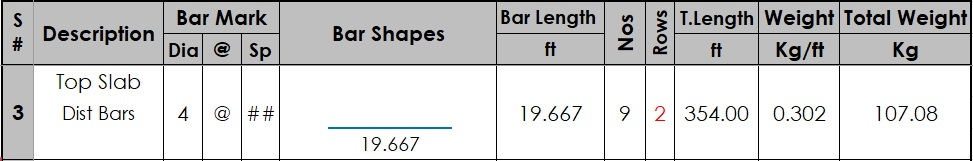Cut Length of Distribution Bars = Length of Culvert – (2 x CC)

Cut Length of Distribution Bars = 20’ – (2 x 0.17’)

Cut Length of Distribution Bars = 20’- 0.34

Cut Length of Distribution Bars = 19.667’

Reinforcement Details of RCC Box Culvert

No’s of Distribution Bars = (Length of Top Slab – 2xCC) / Spacing +1

No’s of Distribution Bars = (7.67’ – 2×0.17) / 1 +1

No’s of Distribution Bars = 7.33 / 1 + 1 = 7.33 + 1

No’s of Distribution Bars = 8.33 = Say 9 No’s (In Single Layer)

Total No’s of Distribution Bars = (Top Layer + Bottom Layer) = 9 + 9 = 18 No’s

Reinforcement Details of RCC Box Culvert

Total Length of Distribution Bars = Cut Length x No’s of Bars

Total Length of Distribution Bars = 19.667’ x 18

Total Length of Distribution Bars = 354.00’

Weight of Distribution Bars = d2/52.896 x Total Length (where d is in # Nos)

Weight of Distribution Bars = 42/52.896 x 354.00

Weight of Distribution Bars = 107.08 Kg

#### 4. Bottom Slab Top Main Bars of RCC Box Culvert: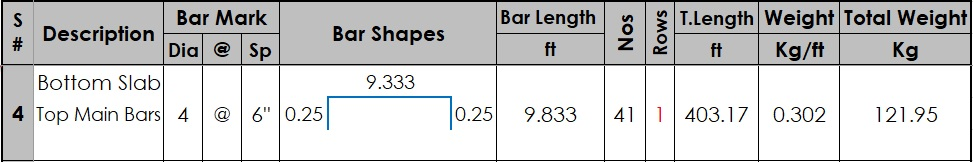Cut Length of Top Mai Bars = Length of Top Slab – (2 x CC) + (2 x Bend Length)

Cut Length of Top Main Bars = 9.67’ – (2 x 0.17’) + (2 x 0.25’)

Cut Length of Top Main Bars = 9.67’- 0.34 + 0.50

Cut Length of Top Main Bars = 9.833’

No’s of Top Main Bars = (Length of Culvert – 2xCC) / Spacing +1

No’s of Top Main Bars = (20’ – 2×0.17) / 0.5 +1

No’s of Top Main Bars = 19.66 / 0.5 + 1 = 39.32 + 1

No’s of Top Main Bars = 40.32 = Say 41 No’s

Total Length of Top Main Bars = Cut Length x No’s of Bars

Total Length of Top Main Bars = 9.833’ x 41

Total Length of Top Main Bars = 403.153

Weight of Top Main Bars = d2/52.896 x Total Length (where d is in # Nos)

Weight of Top Main Bars = 42/52.896 x 403.153

Weight of Top Main Bars = 121.95 Kg

#### 5. Bottom Slab Bottom Main Bars of RCC Box Culvert: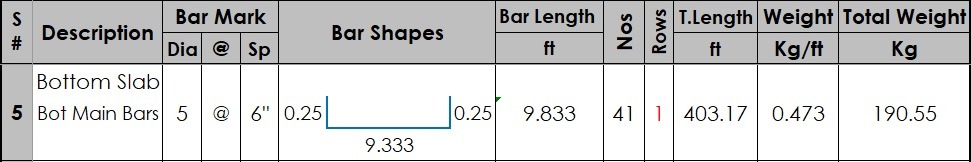Cut Length of Bottom Mai Bars = Length of Top Slab – (2 x CC) + (2 x Bend Length)

Cut Length of Bottom Top Main Bars = 9.67’ – (2 x 0.17’) + (2 x 0.25’)

Cut Length of Bottom Main Bars = 9.67’- 0.34 + 0.50

Cut Length of Bottom Main Bars = 9.833’

No’s of Bottom Main Bars = (Length of Culvert – 2xCC) / Spacing +1

No’s of Bottom Main Bars = (20’ – 2×0.17) / 0.5 +1

No’s of Bottom Main Bars = 19.66 / 0.5 + 1 = 39.32 + 1

No’s of Bottom Main Bars = 40.32 = Say 41 No’s

Total Length of Bottom Main Bars = Cut Length x No’s of Bars

Total Length of Bottom Main Bars = 9.833’ x 41

Total Length of Bottom Main Bars = 403.153

Weight of Bottom Main Bars = d2/52.896 x Total Length (where d is in # Nos)

Weight of Bottom Top Main Bars = 52/52.896 x 403.153

Weight of Bottom Top Main Bars = 190.55 Kg

#### 6. Bottom Slab Distribution Bars of RCC Box Culvert: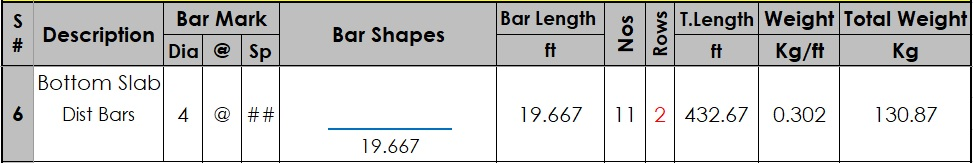Cut Length of Distribution Bars = Length of Culvert – (2 x CC)

Cut Length of Distribution Bars = 20’ – (2 x 0.17’)

Cut Length of Distribution Bars = 20’- 0.34

Cut Length of Distribution Bars = 19.667’

No’s of Distribution Bars = (Length of Bottom Slab – 2xCC) / Spacing +1

No’s of Distribution Bars = (9.67’ – 2×0.17) / 1 +1

No’s of Distribution Bars = 7.33 / 1 + 1 = 9.33 + 1

No’s of Distribution Bars = 10.33 = Say 11 No’s (In Single Layer)

Total No’s of Distribution Bars = (Top Layer + Bottom Layer) = 11 + 11 = 22 No’s

Total Length of Distribution Bars = Cut Length x No’s of Bars

Total Length of Distribution Bars = 19.667’ x 22

Total Length of Distribution Bars = 432.67’

Weight of Distribution Bars = d2/52.896 x Total Length (where d is in # Nos)

Weight of Distribution Bars = 42/52.896 x 432.67

Weight of Distribution Bars = 130.87 Kg

#### 7. Outside Walls Vertical Bars of RCC Box Culvert: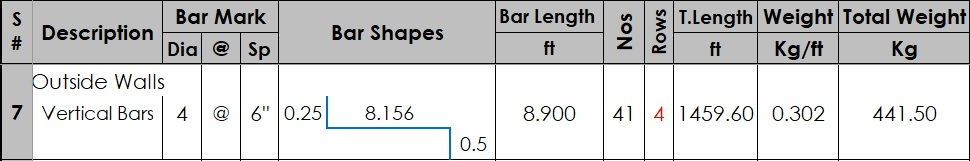Cut Length of Vertical Bars = Length of Wall with Top & Bot Slab – (2 x CC) – (Dia of Bottom Slab Main & Dist Bar) + (Top Bend Length) + (Bot Development Length)

Cut Length of Vertical Bars = 8.583’ – (2 x 0.17’) – (0.09375’) + (0.25’) + (0.5’)

Cut Length of Vertical Bars = 8.583’- 0.34’ – 0.09375’ + 0.25’ + 0.5’

Cut Length of Vertical Bars = 8.90’

No’s of Vertical Bars = (Length of Culvert – 2xCC) / Spacing +1

No’s of Vertical Bars = (20’ – 2×0.17) / 0.5 +1

No’s of Vertical Bars = 19.66 / 0.5 + 1 = 39.32 + 1

No’s of Vertical Bars = 40.32 = Say 41 No’s (In Single Layer)

Total No’s of Vertical Bars = (Total layers of Vertical Bars in Walls are 4) = 41 x 4 = 164 No’s

Total Length of Vertical Bars = Cut Length x No’s of Bars

Total Length of Vertical Bars = 8.90’ x 164

Total Length of Vertical Bars = 1459.60’

Weight of Vertical Bars = d2/52.896 x Total Length (where d is in # Nos)

Weight of Vertical Bars = 42/52.896 x 1459.60

Weight of Vertical Bars = 441.50 Kg

#### 8. Outside Walls Distribution Bars of RCC Box Culvert: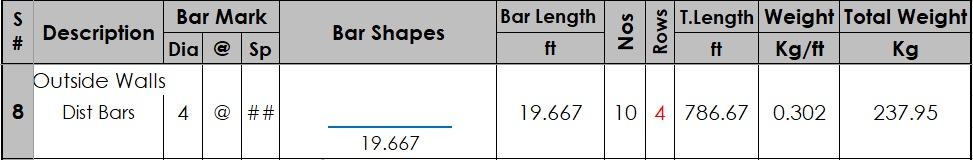Cut Length of Distribution Bars = Length of Culvert – (2 x CC)

Cut Length of Distribution Bars = 20’ – (2 x 0.17’)

Cut Length of Distribution Bars = 20’- 0.34

Cut Length of Distribution Bars = 19.667’

No’s of Distribution Bars in wall = (Length of Wall with top & Bot Slab – 2xCC – Dia of Bottom Slab Main & Dist Bar) / Spacing +1

No’s of Distribution Bars = (8.583’ – 2×0.17 – 0.09375) / 1 +1

No’s of Distribution Bars = 8.14925 / 1 + 1 = 8.14925 + 1

No’s of Distribution Bars = 9.14925 = Say 10 No’s (In Single Layer)

Total No’s of Distribution Bars = (Total layers of Dist Bars in Walls are 4) = 10 x 4 = 40 No’s

Total Length of Distribution Bars = Cut Length x No’s of Bars

Total Length of Distribution Bars = 19.667’ x 40

Total Length of Distribution Bars = 786.67’

Weight of Distribution Bars = d2/52.896 x Total Length (where d is in # Nos)

Weight of Distribution Bars = 42/52.896 x 786.67

Weight of Distribution Bars = 237.95 Kg

#### 9. Concrete Fillet Haunch Bars of RCC Box Culvert: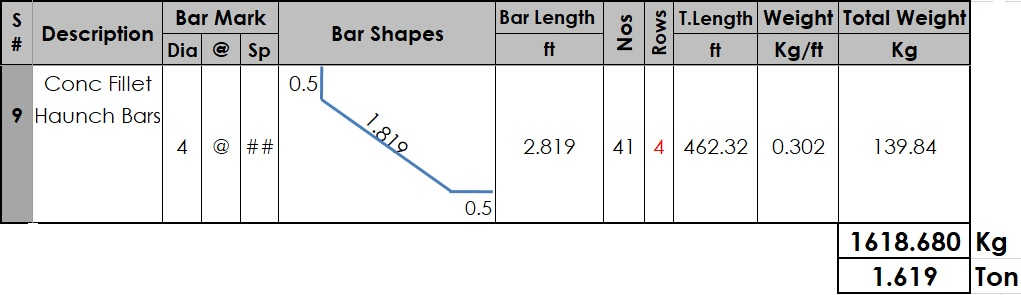As per the Culvert Cross Section;

X = 18” – CC – (#4 bar) = 18” – 2” – 0.5” = 15.5”/12 = 1.2922 = 1.668′

Y = 8” + 10” – CC – (#5 Bar) = 8” + 10” – 2” – 0.625” = 15.375”/12 = 1.2812 = 1.642′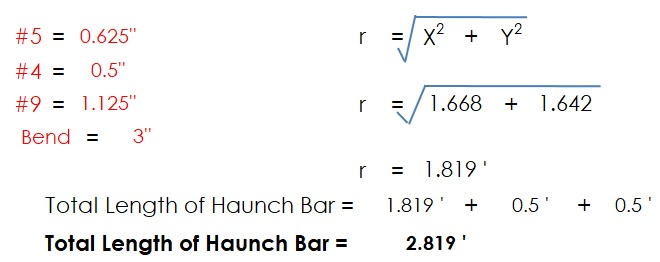No’s of Haunch Bars = (Length of Culvert – 2xCC) / Spacing +1

No’s of Haunch Bars = (20’ – 2×0.17) / 0.5 +1

No’s of Haunch Bars = 19.66 / 0.5 + 1 = 39.32 + 1

No’s of Haunch Bars = 40.32 = Say 41 No’s (In Single Layer)

Total No’s of Haunch Bars = (Total layers of Vertical Bars in Walls are 4) = 41 x 4 = 164 No’s

Total Length of Haunch Bars = Cut Length x No’s of Bars

Total Length of Haunch Bars = 2.819’ x 164

Total Length of Haunch Bars = 462.32’

Weight of Vertical Bars = d2/52.896 x Total Length (where d is in # Nos)

Weight of Vertical Bars = 42/52.896 x 462.32’

Weight of Vertical Bars = 139.84 Kg

#### Total Weight of Reinforcement:

Total Weight of Reinforcement = 97.15 + 151.79 + 107.08 + 121.95 + 190.55 + 130.87 + 441.50 + 237.95 + 139.84

Total Weight of Reinforcement = 1618.68 Kg = 1.61868 Ton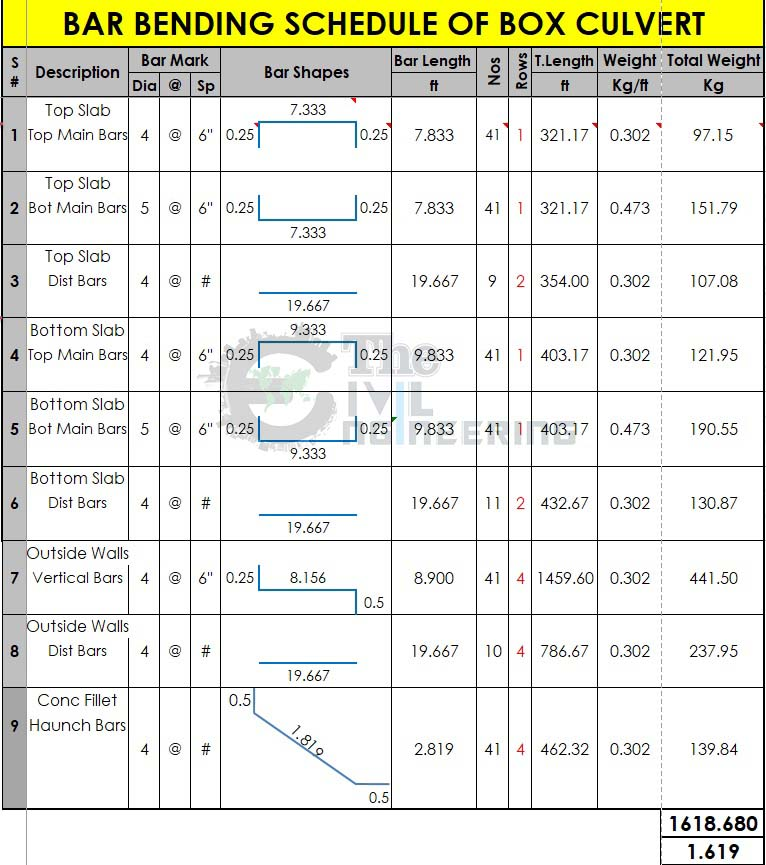(Note: These values may little much different from excel table due to rounding data please ignore it, For Detailed Explanation please watch the above tutorial video)

## Conclusion:

Full article on Bar Bending Schedule for RCC Box Culvert | Box Culvert Quantity Calculation | Advantages of RCC Box Culvert | Box Culvert Estimate | Reinforcement Details of RCC Box Culvert | BBS of Box Culvert. Thank you for the full reading of this article in “The Civil Engineering” platform in English. If you find this post helpful, then help others by sharing it on social media. If you have any question regarding article please tell me in comments.

1.Sohail

• This site is under maintenance i will upload this file soon.

2.Muhammad Junaid khan

Aoa, kindly make a video on rate analysis/ escalation method for BOQ’S.
Thanks

3.zeeshanshahzad

4.Aminullah
5.Prashant Parihar